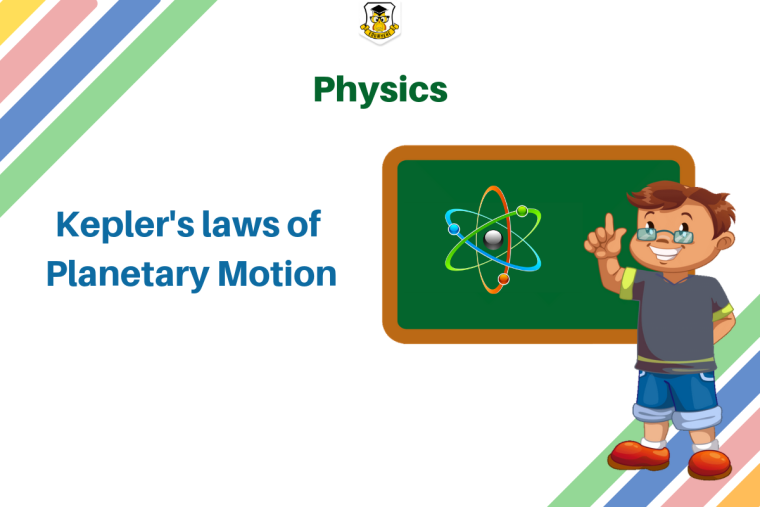## Eduwhere's Concept : Kepler's lawsHere is Eduwhere’s concept of the day.

In astronomy, Kepler's laws of planetary motion are three scientific laws describing the motion of planets around the Sun.

Kepler's three laws of planetary motion can be described as follows:

• The path of the planets about the sun is elliptical in shape, with the centre of the sun being located at one focus. (The Law of Ellipses)

• An imaginary line drawn from the centre of the sun to the centre of the planet will sweep out equal areas in equal intervals of time. (The Law of Equal Areas)

• The ratio of the squares of the periods of any two planets is equal to the ratio of the cubes of their average distances from the sun. (The Law of Harmonies)

1

1583